Spherical zones and caps

Notes by R. Bigoni
(from Note Didattiche)

1. Spherical zone

Given a spherical surface Σ with center O and radius OP with measure R, two parallel planes φ and ψ such that their distance from the center O is smaller than R, intersect Σ in two circumferences.
The region of Σ bounded by these circumferences is called spherical zone Ζ. The circles enclosed by these circumferences are its bases.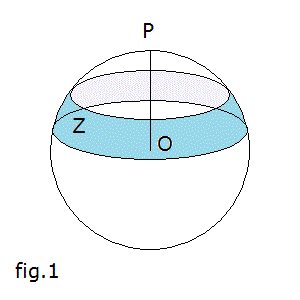To calculate the surface of a spherical zone we can proceed in the following way:

• let's draw from O a straight line OQ perpendicular to OP;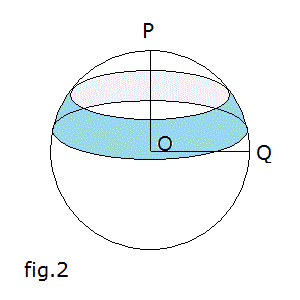• we consider the intersections between Σ, φ and ψ with the plane Π of these two perpendicular lines;
• we assume the straight line OQ as the axis of the abscissas and the line OP as the axis of the ordinates;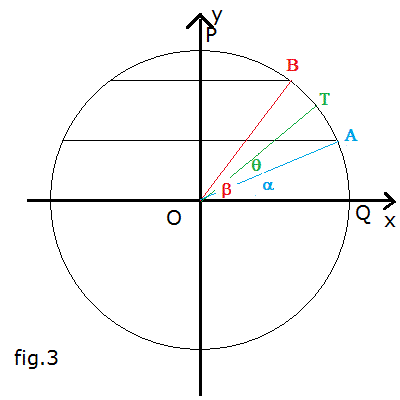• the points A and B are respectively the intersections in the first quadrant between γ and the planes φ and ψ; T is any point of γ;

the radiuses OA, OB and OT form, respectively, the angles α, β and θ with the positive direction of the x axis;

the coordinates of A, B and T are respectively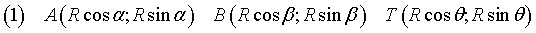• an infinitesimal segment of γ has length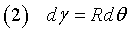• the area S of the zone Z can be conceived as the sum of the lateral surfaces of cylinders of infinitesimal height and base radius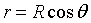with θ from α to β;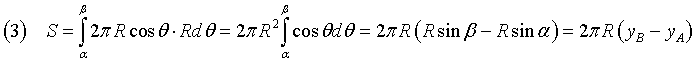• if we represent by δ the distance between the planes φ e ψ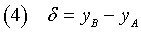we get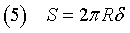2. Spherical segment

With reference to fig. 1, the solid Ω bounded by the spherical zone Z and its bases is called spherical segment.

With reference to fig. 3 and with a method similar to that used for the calculation of S, the volume V of Ω can be calculated as the sum of the volumes of the cylinders with infinitesimal height dy and base radius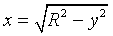with y from yA to yB;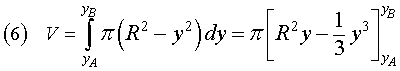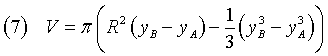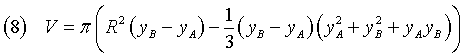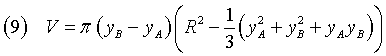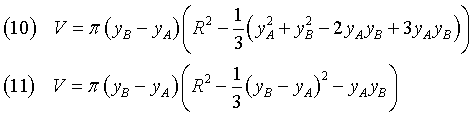From the equation (4) we get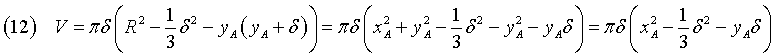Moreover, if we apply the Pythagorean theorem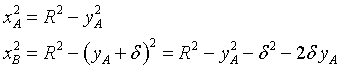and subtract side by side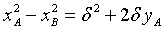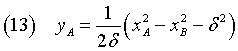If we plug this expression for yA into the equation (12)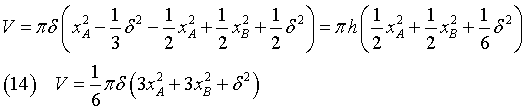3. Spherical cap

If in fig. 1 the plane most distant from the center is tangent to Σ in P, the resulting figure is said spherical cap.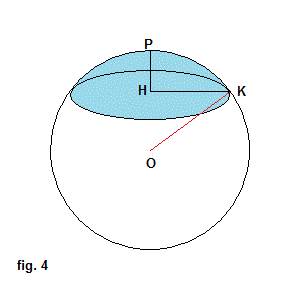In fig. 4, H is the center of the base of the cap, P is its vertex, the segment HK is the radius of the base with measure r, the segment HP is the height of the cap with measure δ and OK is the radius of Σ with measure R.

The equation (5) allows the calculation of the outer surface of the capTh equation (14) with xA=r and xB=0 gives the volume of the cap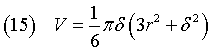If we apply the Pythagorean theorem, we get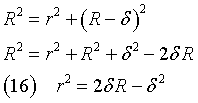and, plugging this expression for r2 into the equation (15), we obtain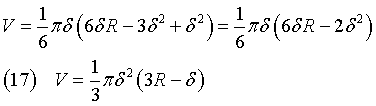3. Visible cap of a spherical surface.

Given a spherical surface Σ with center O and radius R and the point E outside Σ at distance h from it, the portion of Σ observable from E is a cap whose area can be calculated as follows:

• we consider the cone Γ with vertex in E, with lateral surface tangent to Σ and base formed by the set of tangency points;
• we represent the intersections of Σ and Γ with a plane passing through the line OE;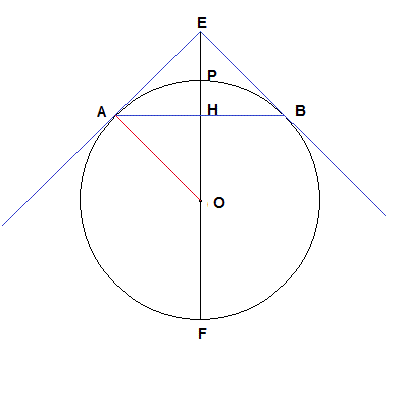the section of Σ is a circumference; the section of Γ is an isosceles triangle with vertices E, A and B;
the height of the triangle lies on the line of the diameter EF, intersects the circumference in P and intersects the base in H;

• the triangle EAO is right-angled in A;
• the triangle EAO is similar to the triangle AHO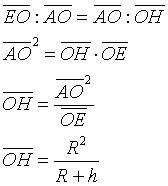• in the cap we have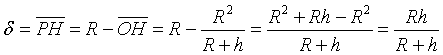• and finally, from the equation (5),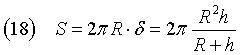The surface of Σ is S=4πR2, hence the fraction of Σ visible from E is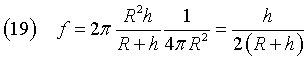last updated: March 16, 2018